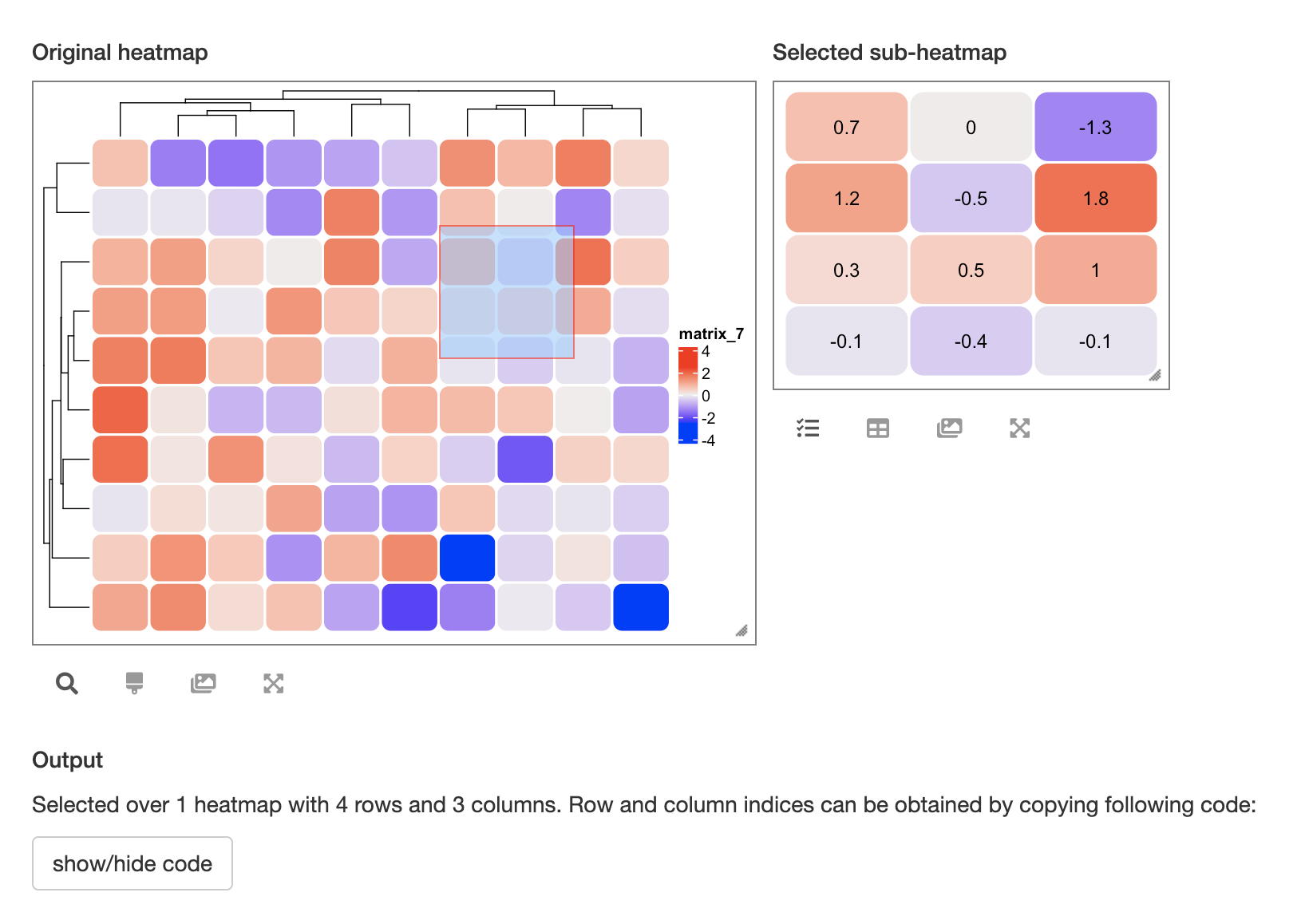# Set cell_fun/layer_fun in InteractiveComplexHeatmap

In the interactive heatmap produced by InteractiveComplexHeatmap package, the sub-heatmap is just a zooming of the main heatmap, which means, if `cell_fun` or `layer_fun` is set in `Heatmap()`, both main heatmap and sub-heatmap will execute it. `cell_fun` and `layer_fun` provides a way to customize the heatmap cells, but when the main heatmap is huge, adding more graphics via `cell_fun`/`layer_fun` apparently is not a good idea. Users may want to suppress `cell_fun`/`layer_fun` (or just draw very simple graphics which will not disturb the reading of the heatmap) in the main heatmap, while only to execute them in the sub-heatmap.

Now in InteractiveComplexHeatmap, there is a new function `is_in_sub_heatmap()` which tells whether the current environment is in main heatmap or in the sub heatmap. This function can be used inside `cell_fun`/`layer_fun` so that users can decide which graphics are only drawn for sub-heatmaps.

In the following example, I defined a `cell_fun` which draws cells as round rectangles, and only in the sub-heatmap, the values in the matrix are added as text labels.

``````library(InteractiveComplexHeatmap)
library(ComplexHeatmap)

m = matrix(rnorm(100), 10)

ht = Heatmap(m, rect_gp = gpar(type = "none"),
show_row_dend = FALSE, show_column_dend = FALSE,
cell_fun = function(j, i, x, y, w, h, fill) {
grid.roundrect(x, y, w, h, r = unit(0.2, "snpc"),
gp = gpar(col = "white", fill = fill, lwd = 2))

if(is_in_sub_heatmap()) {
grid.text(round(m[i, j], 1), x, y)
}
})

htShiny(ht)``````